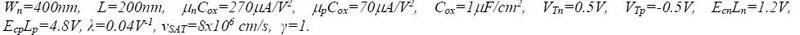# Finding the CMOS transistor width ratio

• diredragon

## Homework Statement

Calculate the ration of ##w_p/w_n## if n and p transistors in CMOS inverter necessary for the least delay time ##t_p## if the circuit is used in a chain of circuits.
a) What is ##w_p## in that circuit if you're given :b) Calculate the maximum short circuit current if ##V_{DD} = 3.3V##.

## Homework Equations

3. The Attempt at a Solution [/B]
The minimum delay time for a CMOS circuit in this scenario is given if ##B## which is ##B=\frac{\frac{w_p}{L_p}}{\frac{w_n}{L_n}}## equals ##B=\sqrt{\frac{Rd_p}{Rd_n}}## where ##R_d## is dynamic resistance of a transistor calculated from a formula ##R_d=\frac{3}{4}\frac{V_{dd}}{I_{dsat}}*(1-5/6λV_{dd})##. So all i need to do is calculate both resistances divide them and get what ##B## equals so i can calculate ##w_p##.
For a N-Mos transistor ##I_{dsat}=1/2*\frac{μ_nC_{ox}w_n}{L_n}*(V_{dd} - V_t)^2## (Just for easier calculation I'm ignoring the short canal and effects of ##E_{cn}##. Putting all into equation and divind the parts which are the same i get:
##\frac{Rd_p}{Rd_n} = 270/70\frac{w_n}{w_p}## and if i use that ##w_p = B*w_n## since the lengths are the same i get ##\frac{Rd_p}{Rd_n} = 270/70\frac{1}{B}## and finally taking a square root and extracting ##B## i get that ##B=1.56##. This makes ##w_p = 624nm##. I have no way of checking if this is correct as no solution is given. Could you check and possibly correct the mistakes i made?

#### Attachments

I don't understand what your doing but if I had to do it Id start with the Miller capacitance of the mos being the dominant factor, I had to look up permutivitty on wiki, maybe that will help as all I knew it from was transformers.

Last edited:
•diredragon